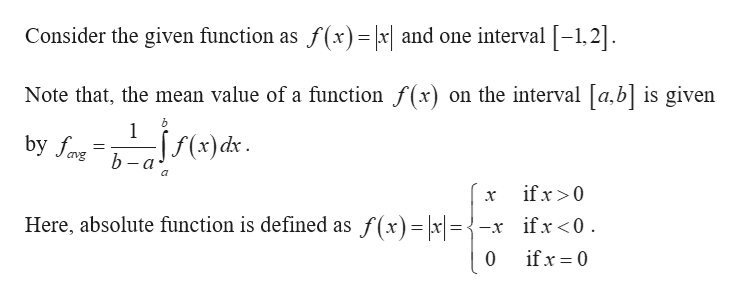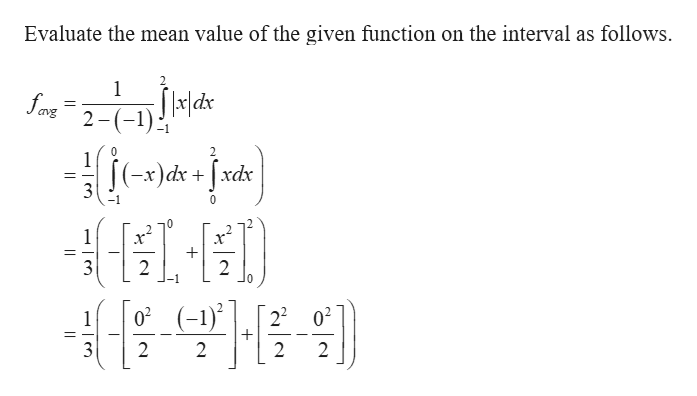# Let f (x) = | x |.The mean value of f on the interval [-1, 2] is ____

Question
1 views

Let f (x) = | x |.
The mean value of f on the interval [-1, 2] is ____

check_circle

star
star
star
star
star
1 Rating
Step 1help_outlineImage TranscriptioncloseConsider the given function as f(x) = \x| and one interval -1,2] Note that, the mean value of a function f(x) on the interval [a,b] is given 1 by fa ;ff(x)ds b-а- ifx >0 х Here, absolute function is defined as f(x)= |x =- ifx<0 if x 0 0 fullscreen
Step 2help_outlineImage TranscriptioncloseEvaluate the mean value of the given function on the interval as follows. avg 2 )dxd 3 -1 0 х* 1 2 2 02 (-) 22 02 1 2 2 2 2 fullscreen

### Want to see the full answer?

See Solution

#### Want to see this answer and more?

Solutions are written by subject experts who are available 24/7. Questions are typically answered within 1 hour.*

See Solution
*Response times may vary by subject and question.
Tagged in

### Integration